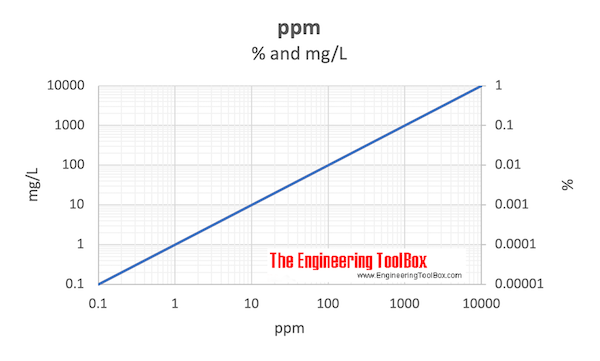Engineering ToolBox - Resources, Tools and Basic Information for Engineering and Design of Technical Applications!

# Parts per Million - ppm

## ppm - or parts per million - is commonly used as a unit of concentration.

Parts per million - ppm - is commonly used as a dimensionless measure of small levels (concentrations) of pollutants in air, water, body fluids, etc.

Parts per million is the molar mass, volume or mass ratio between the pollutant component and the solution. ppm is defined as

ppm = 1,000,000 c / s

= 106 c / s               (1)

where

c = molar mass, volume or mass of the solute component (mole, m3, ft3, kg, lbm)

s = molar mass, volume or mass of the solution (mole, m3, ft3, kg, lbm)

In the metric system ppm for mass can be expressed in terms of milligram versus kg where

• 1 mg/kg = 1 part per million

ppm can be also be expressed as

• 1 ppm = 10-6 = 0.0001 % = 0.001 ‰
• 1 000 ppm = 0.1 %
• 10 000 ppm = 1%Alternatively - mass related units to measure very small concentration levels:

• ppb - parts per billion (1 / 1,000,000,000 or 10-9)
• ppt - parts per trillion (1 / 1,000,000,000,000 or 10-12)
• ppq - parts per quadrillion (1 / 1,000,000,000,000,000 or 10-15)

An alternatively mass related unit to measure larger concentration levels is weight percent which can be expressed as

weight percent = 100 mc / ms                                  (2)

### ppm vs. Mass per Unit Volume

The concentration of a component can be measured as mass per unit volume - like mg/liter, mg/cm3 etc.

Weight of substance added to one unit volume of water to give one part per million (ppm)

1 ppm

= 2.72 pounds per acre-foot

= 1,233 grams per acre-foot

= 1.233 kilograms per acre-foot

= 0.0283 grams per cubic foot

= 0.0000624 pounds per cubic foot

= 0.0038 grams per US gallon

= 0.058419 grains per US gallon

= 0.07016 grains per Imperial gallon

= 1 milligram per liter (mg/L)

= 1 microlitre ( μL ) per liter

= 0.001 gram per litre

= 8.345 pounds per million gallons of water

#### Example - Volume Concentration of Carbon Dioxide in Air

The concentration of carbon dioxide in air is aprox. 400 ppm. The volume of carbon dioxide in one 1 m3 of air can be calculated by modifying (1) to

c = ppm s / 106

= (400 ppm) (1 m3) / 106

=  0.0004 m3/m3

### Percent by Volume

Volume percent can be expressed as volume per unit volume:

percent by volume = 100 vc /(vc + vs)                               (3)

where

vc = volume component

vs = volume solvent

### Molarity

Molarity is the number of moles of solute (substance of interest - pollution, etc.) dissolved in one liter (volume) of the solution.

### Molality

Molality is the number moles of solute divided by kilograms of solvent.

## Related Topics

• Basics - The SI-system, unit converters, physical constants, drawing scales and more.

## Engineering ToolBox - SketchUp Extension - Online 3D modeling!

Add standard and customized parametric components - like flange beams, lumbers, piping, stairs and more - to your Sketchup model with the Engineering ToolBox - SketchUp Extension - enabled for use with the amazing, fun and free SketchUp Make and SketchUp Pro .Add the Engineering ToolBox extension to your SketchUp from the SketchUp Pro Sketchup Extension Warehouse!

Translate

## Privacy

We don't collect information from our users. Only emails and answers are saved in our archive. Cookies are only used in the browser to improve user experience.

Some of our calculators and applications let you save application data to your local computer. These applications will - due to browser restrictions - send data between your browser and our server. We don't save this data.

## Citation

• Engineering ToolBox, (2008). Parts per Million - ppm . [online] Available at: https://www.engineeringtoolbox.com/ppm-d_1039.html [Accessed Day Mo. Year].

Modify access date.

. .

#### Scientific Online Calculator3 30